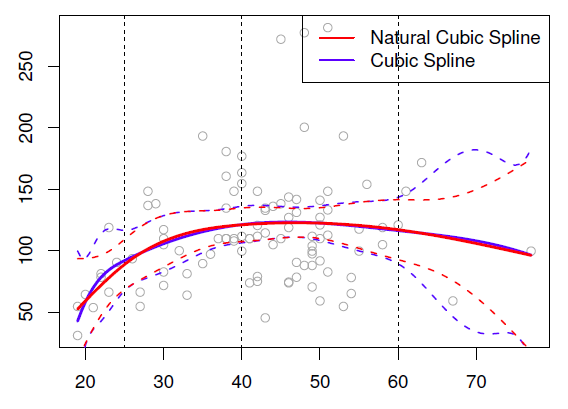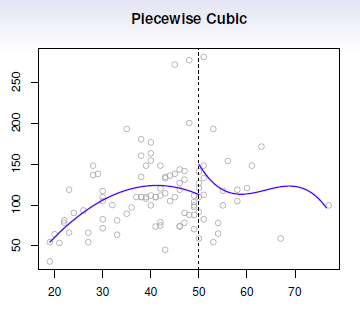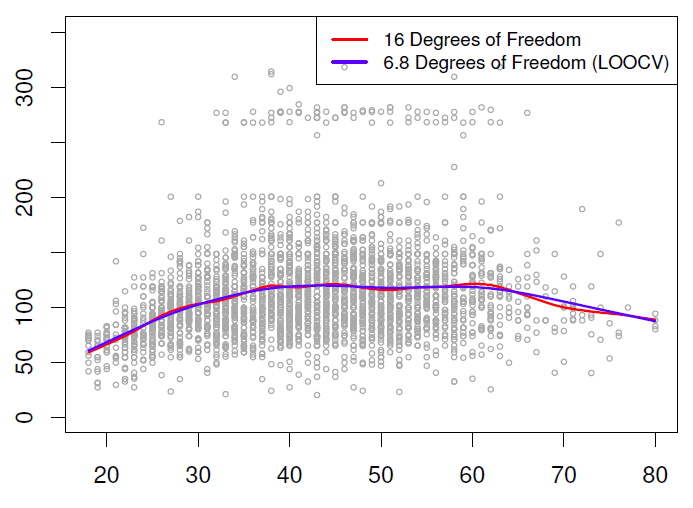# Statistics - Cubic spline

Cubic splines is a step cubic piecewise polynomial in the left and the right and continuous at the knot.

There's the following constraints:

• The first derivatives continues at the knot,
• and the second derivatives continues at the knot.

We couldn't make the third derivative continuous, because then it would just be a global cubic polynomial. It's believed that a cubic spline, the discontinuity which exists in the third derivative is not detectable by the human eye.

The degree of Statistics - Piecewise polynomials is 2 because it's a third degree polynomial.

## Definition / Representation

A cubic spline with knots at $\xi_k$ with $k = 1, \dots, K$ is a piecewise cubic polynomial with continuous derivatives up to order 2 at each knot.

As a linear spline, this model can be represented with truncated power basis (functions|expansion|transformation) in the original variables

$$y_i = \beta_0 + \beta_1.b_1(x_i) + \beta_2.b_2(x_i) + \dots + \beta_{K+3}b_{K+3}(x_i) + \epsilon_i$$

where the $b_k$ are basis functions and are:

• the variable itself. The first basis functions is just the variable itself

$$\begin{array}{rll} b_1(x_i) & = & x_i \\ \end{array}$$

• the variable squared

$$\begin{array}{rll} b_2(x_i) & = & x_i^2 \\ \end{array}$$

• the variable cubed

$$\begin{array}{rll} b_3(x_i) & = & x_i^3 \\ \end{array}$$

• and additional variables that are a collection of truncated basis transformation functions at each of the knots. They are called truncated power functions and this one is to power 3 (cubic).

$$\begin{array}{rll} b_{k+3}(xi) & = & (xi - \xi_k)_+^3 \text{ where } k = 1, \dots, K \\ \text{where the ()+ means positive part. i.e. } (xi - \xi_k)^3_+ & = & \left\{ \begin{matrix} (xi - \xi_k)^3 & \text{ if } x_i > \xi_k & \\ 0 & \text{Otherwise} & \text{It's not allowed to go negative.} \end{matrix} \right. \end{array}$$

## Plot

At the knot, the basis function:

• is 0
• got 0 first derivative and 0 second derivative.

Therefore, when we add it, we don't change the derivatives of the function and so has the required continuity of a cubic spline.

## Degree of freedom

A cubic spline with K knots has K plus 4 parameters or degrees of freedom.

Recommended PagesR - Spline

Fitting splines in R is easy: bs(x, ...) for any degree splines (one: linear spline, three: cubic spline and ns(x, ...) for natural cubic splines, in package splines.Statistics - Natural (Cubic) Spline

A natural cubic spline is a cubic spline where two extra constraints have been added at the boundaries (on each end). The goal of this constraints is to avoid as for global cubic polynomial that the tail...Statistics - Piecewise polynomials

Piecewise polynomials generalize the idea of piecewise constants. The idea try to get rid of the global polynomial because it's global and not local. Instead of having asingle polynomial over the whole...Statistics - Smoothing (cubic) spline

A smoothing spline is a way of fitting splines without having to worry knots. They are a little bit more challenging mathematically as others splines and approaches the problem from a completely different...Statistics - Splines

A spline is a piecewise polynomial model: Splines have the “maximum” amount of continuity over all knots function.